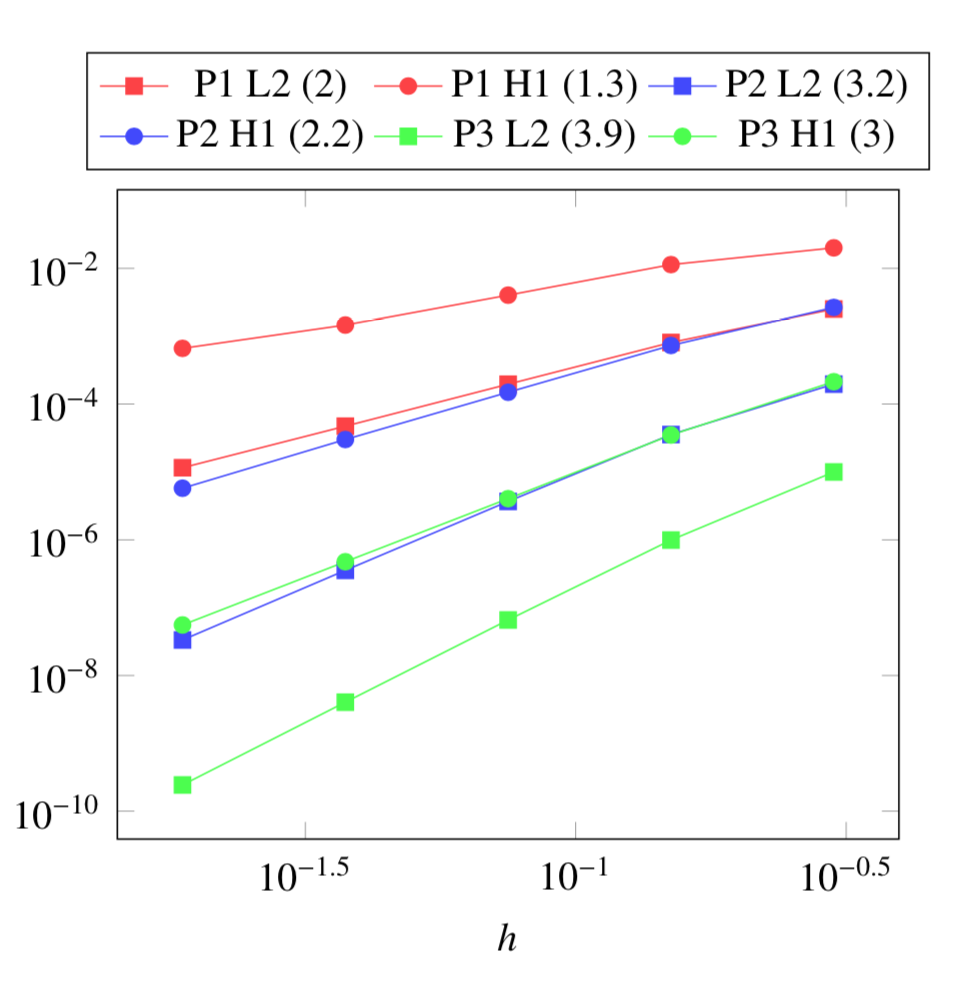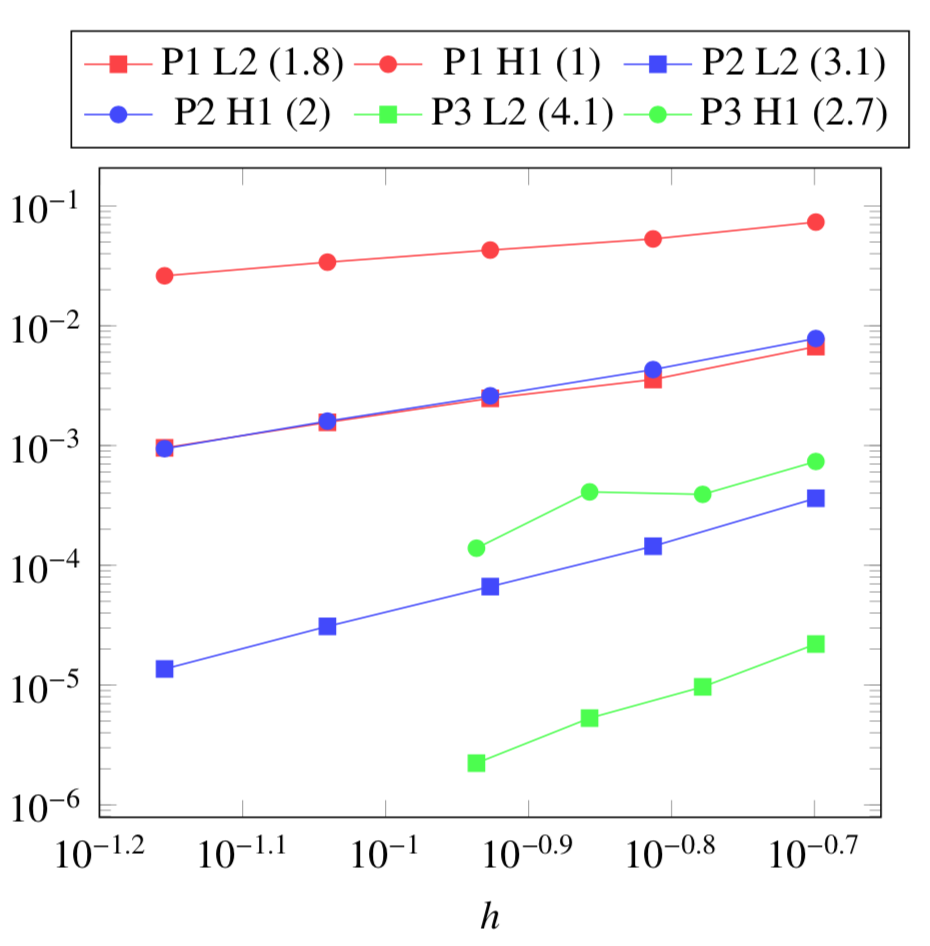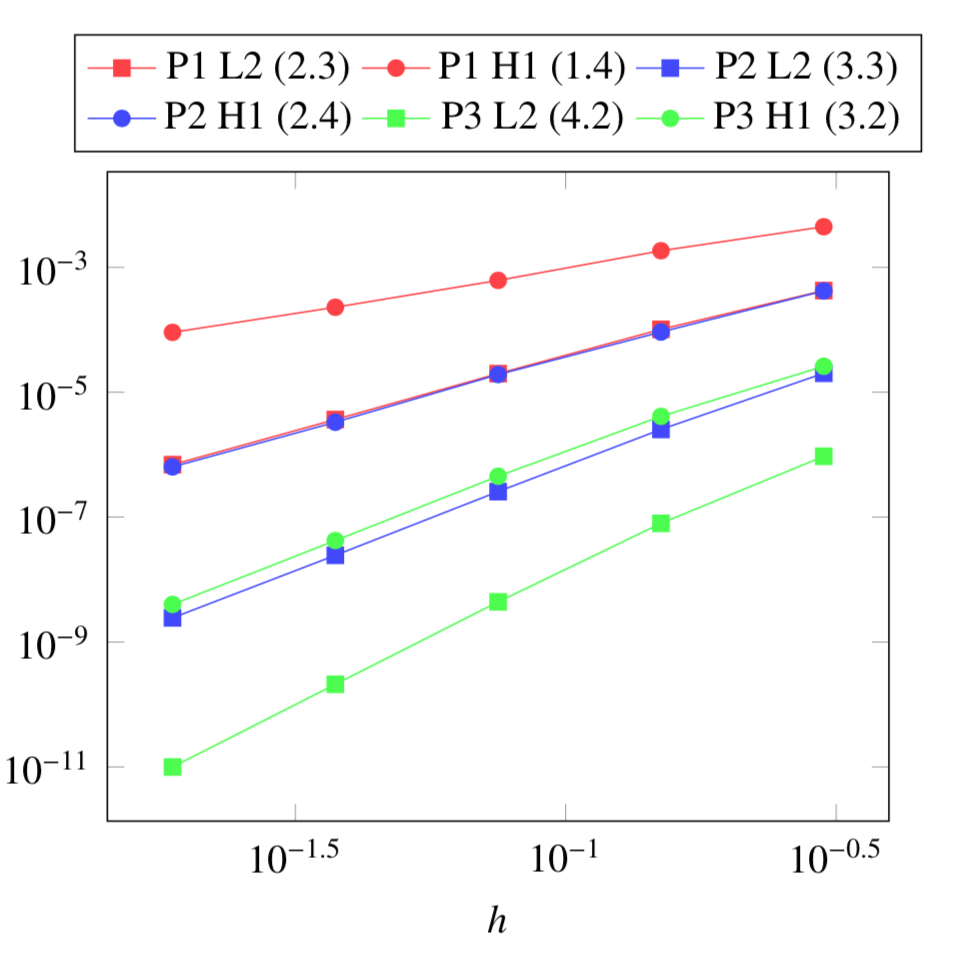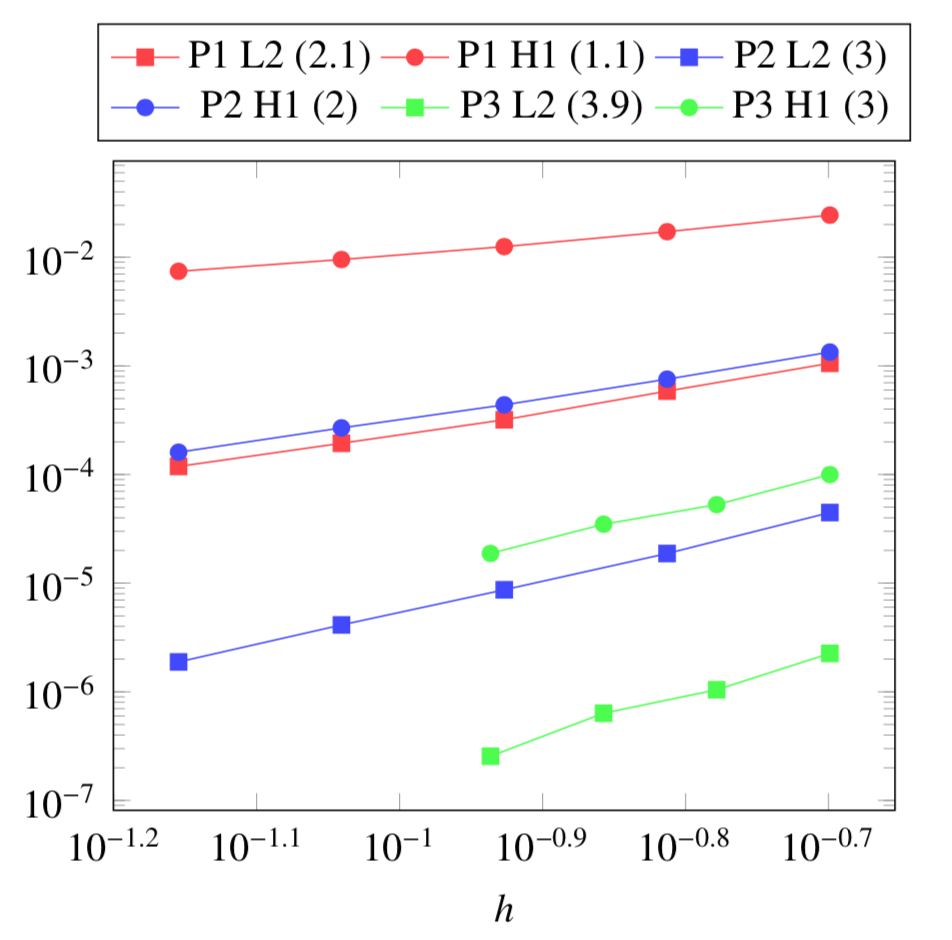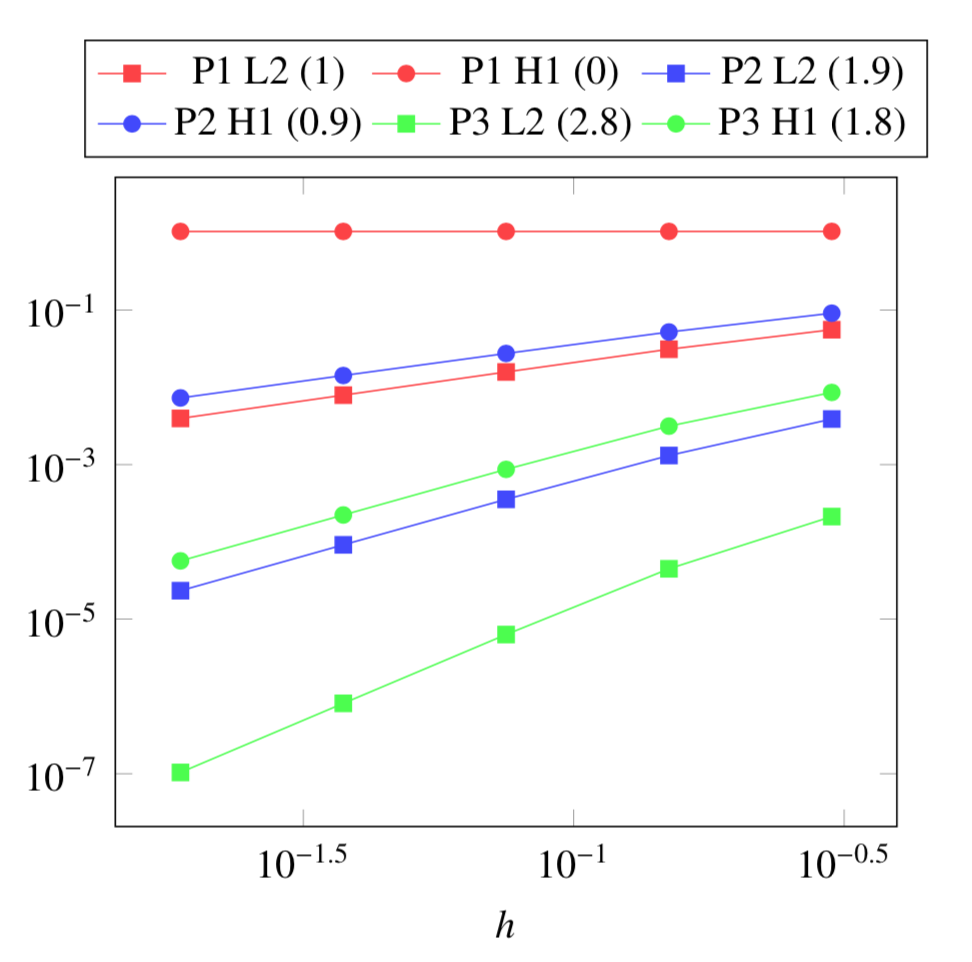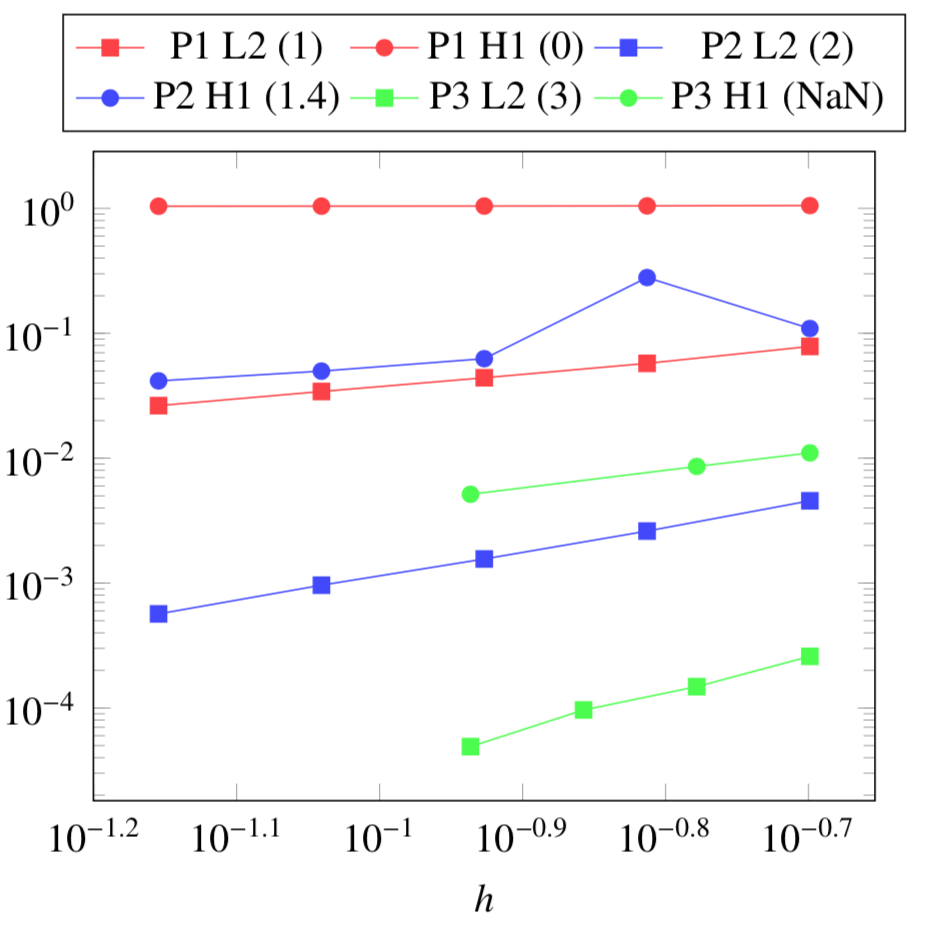# QuarterTurn

In this example, we will estimate the rise in temperature due to Joules losses in a stranded conductor. An electrical potential $V_0$ is applied to the entry/exit of the conductor which is also cooled by a force flow.
The geometry of the conductor is choosen as to have an analytical expression for the temperature.

## 1. Running the case

The command line to run this case in 2D is

``mpirun -np 4 feelpp_toolbox_thermoelectric --case "github:{path:toolboxes/thermoelectric/cvg}" --case.config-file 2d.cfg``

The command line to run this case in 3D is

``mpirun -np 4 feelpp_toolbox_thermoelectric --case "github:{path:toolboxes/thermoelectric/cvg}" --case.config-file 3d.cfg``

## 2. Geometry

The conductor consists in a rectangular cross section torus which is somehow "cut" to allow for applying electrical potential. The conductor is cooled with a force flow along its cylindrical faces.+ In 2D, the geometry is as follow: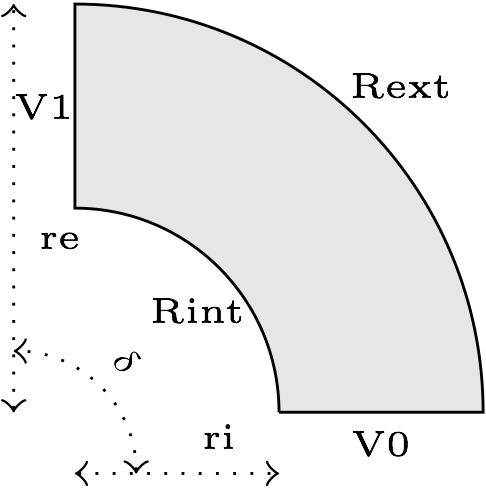In 3D, this is the same geometry, but extruded along the z axis.

## 3. Input parameters

Name Description Value Unit

$r_i$

1

$m$

$r_e$

2

$m$

$\delta$

angle

$\pi/2$

$rad$

$V_D$

electrical potential

9

$V$

$h_i$

internal transfer coefficient

$60e3$

$W\cdot m^{-2}\cdot K^{-1}$

$T_{wi}$

internal water temperature

303

$K$

$h_e$

external transfer coefficient

$58e3$

$W\cdot m^{-2}\cdot K^{-1}$

$T_{we}$

external water temperature

293

$K$

### 3.1. Model & Toolbox

• This problem is fully described by a Thermo-Electric model, namely a poisson equation for the electrical potential $V$ and a standard heat equation for the temperature field $T$ with Joules losses as a source term.

• toolbox: thermoelectric

### 3.2. Materials

Name Description Marker Value Unit

$\sigma$

electric conductivity

omega

$4.8e7$

$S.m^{-1}$

### 3.3. Boundary conditions

The boundary conditions for the electrical probleme are introduced as simple Dirichlet boundary conditions for the electric potential on the entry/exit of the conductor. For the remaining faces, as no current is flowing througth these faces, we add Homogeneous Neumann conditions.

Marker Type Value

V0

Dirichlet

0

V1

Dirichlet

$V_D$

Rint, Rext, top*, bottom*

Neumann

0

As for the heat equation, the forced water cooling is modeled by robin boundary condition with $Tw$ the temperature of the coolant and $h$ an heat exchange coefficient.

Marker Type Value

Rint

Robin

$h_i(T-T_{wi})$

Rext

Robin

$h_e(T-T_{we})$

V0, V1, top*, bottom*

Neumann

0

*: only in 3D

## 4. Outputs

The main fields of concern are the electric potential $V$, the temperature $T$ and the current density $\mathbf{j}$ or the electric field $\mathbf{E}$. // presented in the following figure.

## 5. Verification Benchmark

The analytical solutions are given by:

\begin{align*} V&=\frac{V_D}{\delta}\theta=\frac{V_D}{\delta}\operatorname{atan2}(y,x)\\ \mathbf{E}&=\left( -\frac{V_D}{\delta}\frac{y}{x^2+y^2}, \frac{V_D}{\delta}\frac{x}{x^2+y^2}\right)\\ T&=A\log(r)^2+B\log(r)+C=A\log\left(\sqrt{x^2+y^2}\right)^2+B\log\left(\sqrt{x^2+y^2}\right)+C\\ A&=-\frac{\sigma}{2k}\left(\frac{V_D}{\delta}\right)^2\\ B&=\frac{B_e-B_i}{D}\\ C&=\frac{C_e-C_i}{D}\\ B_e&=2T_{we}\delta^2h_eh_ikr_er_i + V_D^2h_eh_ir_er_i\sigma\log(r_e)^2 + V_D^2h_ikr_i\sigma\log(r_e^2)\\ B_i&=2T_{wi}\delta^2h_eh_ikr_er_i + V_D^2h_eh_ir_er_i\sigma\log(r_i)^2 - V_D^2h_ekr_e\sigma\log(r_i^2)\\ C_e&=(h_er_e\log(r_e) + k)(2T_{wi}\delta^2h_ikr_i + V_D^2h_ir_i\sigma\log(r_i)^2 - V_D^2k\sigma\log(r_i^2))\\ C_i&=(h_ir_i\log(r_i) - k)(2T_{we}\delta^2h_ekr_e + V_D^2h_er_e\sigma\log(r_e)^2 + V_D^2k\sigma\log(r_e^2))\\ D&=2\delta^2k(h_eh_ir_er_i\log(r_e) - h_eh_ir_er_i\log(r_i) + h_ekr_e + h_ikr_i) \end{align*}

We will check if the approximations converge at the appropriate rate:

• k+1 for the $L_2$ norm for $V$ and $T$

• k for the $H_1$ norm for $V$ and $T$

• k for the $L_2$ norm for $\mathbf{E}$ and $\mathbf{j}$

• k-1 for the $H_1$ norm for $\mathbf{E}$ and $\mathbf{j}$